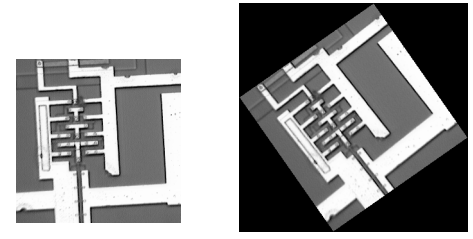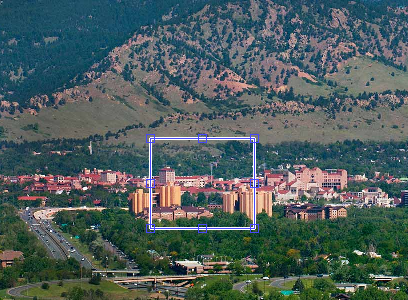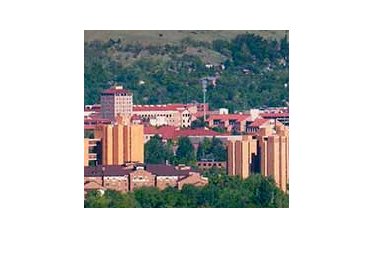# Geometric Transformations

#### Interpolation

Interpolation is the process by which the software resamples the image data to determine values between defined pixels. For example, if you resize an image so it contains more pixels than it did originally, the software obtains values for the additional pixels through interpolation.

The Image Processing Toolbox provides three interpolation methods:

•Nearest neighbor interpolation
•Bilinear interpolation
•Bicubic interpolation

The interpolation methods all work in a fundamentally similar way. In each case, to determine the value for an interpolated pixel, you find the point in the input image that the output pixel corresponds to. You then assign a value to the output pixel by computing a weighted average of some set of pixels in the vicinity of the point. The weightings are based on the distance each pixel is from the point.

The methods differ in the set of pixels that are considered:

•For nearest neighbor interpolation, the output pixel is assigned the value of the pixel that the point falls within. No other pixels are considered.
•For bilinear interpolation, the output pixel value is a weighted average of pixels in the nearest 2-by-2 neighborhood.
•For bicubic interpolation, the output pixel value is a weighted average of pixels in the nearest 4-by-4 neighborhood.

The number of pixels considered affects the complexity of the computation. Therefore the bilinear method takes longer than nearest neighbor interpolation, and the bicubic method takes longer than bilinear. However, the greater the number of pixels considered, the more accurate the effect is, so there is a tradeoff between processing time and quality

#### Image Resizing

The toolbox function imresize changes the size of an image using a specified interpolation method. If you do not specify an interpolation method, the function uses nearest neighbor interpolation. You can use imresize to resize an image by a specific magnification factor. To enlarge an image, specify a factor greater than 1. For example, the command below doubles the number of pixels in X in each direction.

To reduce an image, specify a number between 0 and 1 as the magnification factor. You can also specify the actual size of the output image. The command below creates an output image of size 100-by-150.

If the specified size does not produce the same aspect ratio as the input image has, the output image will be distorted. If you reduce the image size and use bilinear or bicubic interpolation,imresize applies a lowpass filter to the image before interpolation. This reduces the effect of Moiré patterns, ripple patterns that result from aliasing during resampling. Note, however, that even with lowpass filtering, the resizing operation can introduce artifacts, because information is always lost when you reduce the size of an image. imresize does not apply a lowpass filter if nearest neighbor interpolation is used, unless you explicitly specify the filter. This interpolation method is primarily used for indexed images, and lowpass filtering is not appropriate for these images.

#### Image RotationThe imrotate function rotates an image, using a specified interpolation method and rotation angle. If you do not specify an interpolation method, the function uses nearest neighbor interpolation. You specify the rotation angle in degrees. If you specify a positive value, imrotate rotates the image counterclockwise; if you specify a negative value, imrotate rotates the image clockwise.

In order to include the entire original image, imrotate pads the outside with
0’s. This creates the black background in J and results in the output image
being larger than the input image.

#### Image Cropping

The function imcrop extracts a rectangular portion of an image. You can specify the crop rectangle through input arguments, or select it with a mouse. If you call imcrop without specifying the crop rectangle, the cursor changes to a cross hair when it is over the image. Click on one corner of the region you want to select, and while holding down the mouse button, drag across the image. imcrop draws a rectangle around the area you are selecting. When you release the mouse button, imcrop creates a new image from the selected region. If you do not provide any output arguments, imcrop displays the image in a
new figure.